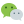# 二进制小数

``````3x10^0 + 1x10^-1 + 2x10^-2 + 5x10^-3
``````

``````3x10^0 + 1/10 + 2/10^2 + 5/10^3
``````

``````3.125（十进制）

3     / 2 = 1    --> 1
余 1    --> 1
0.125 x 2 = 0.25 --> 0
0.25  x 2 = 0.5  --> 0
0.5   x 2 = 1    --> 1

==> 11.001（二进制）
``````

11.001 就是 3.125 的二进制小数形式，同时它也可以做如下拆解：

``````1x2^1 + 1x2^0 + 0x2^-1 + 0x2^-2 + 0x2^-3

= 2 + 1 + 0 + 0 + 0.125
= 3.125
``````

# 定点数

``````0000 0000 0000 0011 0010 0000 0000 0000
``````

# 浮点数

## 标准

``````v = (-1)^s * 2^e * m

v: 浮点数具体值
s: 符号位，即正负号，0 为正，1 为负
m: 有效数，也叫尾数，可以类比科学计数法前面的有效数字
另外还有一个小数位 f, m = 1 + f
e: 指数位，即 2 的多少次方
``````

``````(1)   (8)          (23)          : 位数
0 00000011 0010000000000000000000
s e        f
``````

• 第一位为符号位 s；
• 后 8 位为指数位 e；
• 最后 23 位为小数位 f；

64 位的规则是：

• 第一位是符号位 s；
• 后 11 位是指数 e；
• 最后 52 位是有效数字 m；

## 综合举例

``````3.125
= 0b11.001
= 0b1.1001 x 2^1

s = 0

e = 1
=> 1 + 127
=  128
=  0b10000000

m = ob1.1001
f = m - 1
= 0b0.1001
=> 0b10010000000000000000000

==>
0 10000000 10010000000000000000000
``````

``````1 01111111 00100000000000000000000

s = 1
=> -1

e = 0b01111111
= 127
=> 127 - 127
= 0

f = 0b0.001
m = 1 + f
= 0b1.001

v = -1 x 0b1.001 x 2^0
= 0b-1.001
= -1.125

==> -1.125
``````

## 精度

``````log(2^24) = 7.22
log(2^53) = 15.95
``````

## “浮”的原因

### 评论：微信公众号：程序骑士